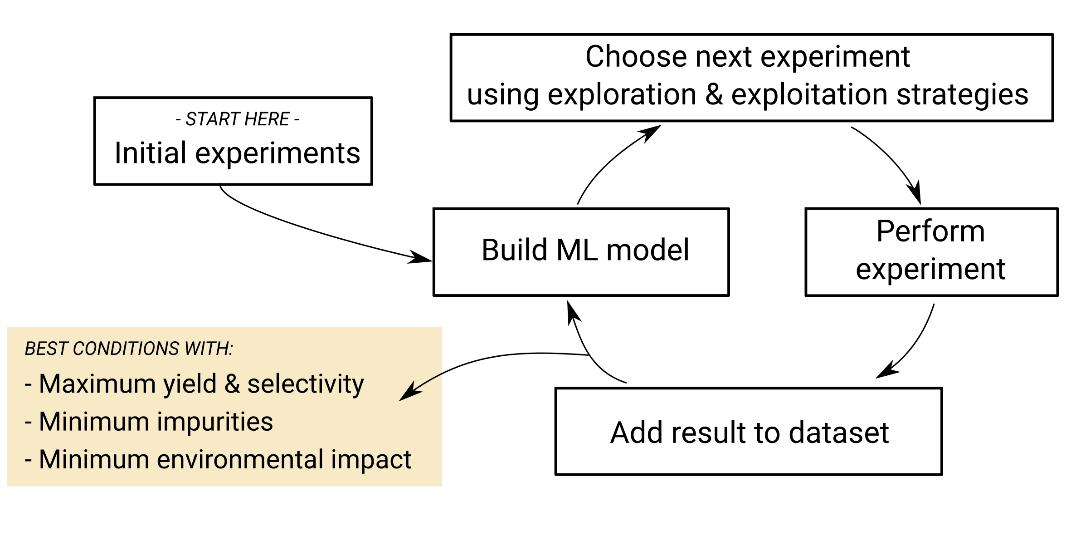20 February 2023

# Bayesian optimisation: Using AI to choose your next experiment

By Thomas Galeandro-Diamant, Director of Products and Services

When chemists want to optimise a chemical reaction or a formulation, the most common method they use is “trial and error”. This involves trying many combinations of parameters, chosen using chemistry knowledge and intuition, until the result meets their requirements. Each experiment brings new knowledge that is useful to design the next experiments. However, trial and error is a slow methodology that it is limited to a few parameters because the number of experiments grows exponentially with the number of parameters, often fails to yield satisfactory results, and can trap chemists in a “local optimum” from where it is difficult to improve.

Some chemists use a technique called “Design of Experiments” (DoE) to save time and increase their probability of success. It involves designing a set of experiments with a computer program, using mathematical techniques that intelligently vary many parameters simultaneously, in order to learn as much as possible from the chemical system. The experiments are then performed and their results are used to build a statistical model, often either linear or polynomial. This statistical model then predicts the theoretically optimal experiment, and the chemist then performs this experiment to confirm that it is, indeed, optimal. Although DoE can be a helpful technique and be faster than trial and error, it is difficult to master and requires user training and expertise. Furthermore, it is limited to a few parameters because the number of experiments grows exponentially with the number of parameters. Hence, it is rarely appropriate for use in the industry, although many chemists know of its existence.

More recently, a technique called “Bayesian optimisation” has been shown to perform better than trial and error and DoE and has gained significantly in popularity in both chemistry1 and formulation2 laboratories. To illustrate the methodology, optimising a chemical reaction or formulation using Bayesian optimisation would involve the following steps.Step one

Define your objectives (e.g. maximise the yield and selectivity of a chemical reaction or find a formulation that has the right viscosity and a high stability) and your constraints (e.g. a minimum and a maximum value for each numerical parameter or some numerical dependencies between several parameters).

Step two

If you have already performed a number of experiments, they will constitute your initial dataset. If you haven’t, an initial experiment will be picked at random by the program, respecting the constraints defined at step one. Perform this experiment. It will constitute your initial dataset.

Step three

The dataset is used to build a machine learning model called a “Gaussian process”. This Gaussian Process model is particularly good at estimating the uncertainty of its predictions, which will be useful for the next step. This uncertainty will be smaller around the experiments contained in the dataset, and higher in areas of the parameter space that haven’t yet been explored. For example, if you have only performed chemical reactions between 30°C and 50°C, the yield predicted by the Gaussian process at 80°C will have a very high uncertainty.

Step four

The Gaussian process model is used to compute the “acquisition function”, which represents how interesting each possible next experiment is. The value of the acquisition function usually depends on how close the predicted result is to the objectives defined at step one (this is called the “Exploitation” strategy) and how large the uncertainty of the Gaussian process prediction for the possible experiment is (this is called the “Exploration” strategy).

Step five

The program will search the experiment for which the value of the Acquisition Function is highest. This is your next experiment.

Step six

Perform this new experiment in a laboratory.

Step seven

Add the new experiment, its parameters and results to the dataset. Once you have reached the objectives defined at step one, you are done. Otherwise, you go back to step three.

Why is Bayesian optimisation such an interesting technique for chemists and formulators?

• It does not need any data (past experiments) to start...
• ...but if you have some data, it can fully leverage it
• It gets more intelligent at each iteration (because the dataset gets larger and the Gaussian Process model is able to make more accurate predictions)
• It generates new ideas (i.e. proposes experiments you wouldn’t have thought of)
• It is able to take into account multiple objectives
• It is able to take into account your constraints

Overall, Bayesian optimisation is a very data-efficient method, meaning that you can reach your objectives in a minimum number of experiments. In most cases, this number of experiments is significantly lower than with a DoE, especially when there are many parameters to adjust.

These significant benefits are the reason we chose Bayesian optimisation as the technique behind our SmartChemistry® platform for optimising chemical reactions and formulations. For a practical example of how Bayesian optimisation works in SmartChemistry®, contact us today to access a demo.

References:

 Shields et al., Nature 2021, 590, 89-96

 Narayanan et al., Mol. Pharmaceutics 2021, 18, 3843-3853